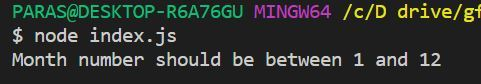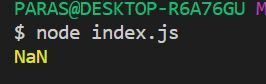Related Articles

# JavaScript Number NaN Property

• Last Updated : 23 Jul, 2020

In JavaScript, NaN stands for Not a Number. It represents a value which is not a valid number. It can be used to check whether a number entered is a valid number or not a number. To assign a variable to NaN value, we can use one of the two following ways.

`var a = NaN`
`var a = Number.NaN`

Example: In this example, we will use JavaScript Number NaN Property.

 `var` `monthNumber = 14;`` ` `if` `(monthNumber < 1 || monthNumber > 12) {`` ` `    ``// Assigning monthNumber NaN as ``    ``// month number is not valid``    ``monthNumber = Number.NaN;`` ` `    ``console.log(``"Month number should be"``                ``+ ``" between 1 and 12"``);``}``else` `{``    ``console.log(monthNumber);``}`

Output:We will see some examples of operations that return NaN.

Example 1: Whenever we try to parse a string or “undefined” to an int, it returns NaN.

 `console.log(parseInt(``"higeeks"``));`

Output:Example 2: Whenever we try to find square root of a negative number using Math.sqrt function, it returns NaN.

 `console.log(Math.sqrt(-1));`

Output:Example 3: Whenever we try to make on operation on NaN, it returns NaN.

 `console.log(5 + NaN);`

Output:Example 4: Any indeterminate form also returns NaN.

 `console.log(0 * Infinity)`

Output:Example 5: Any operation other than addition on a string also results in NaN.

 `console.log(``"hi"``/5)`

Output:My Personal Notes arrow_drop_up